Next: ADC Non-linearities Up: PSPC Gain Previous: Method of Determination

### Spatial Variations

The two main sources of spatial variations in the gain of PSPCs are:

• mechanical inaccuracies of the grid system leading to different electrical field strengths and hence different gains
• the anode wire grid causing a ``binning'', with a period of the wire distance.

(Both effects also give rise to a nonlinearity in the position measurement, see also Sect. 3.5.3).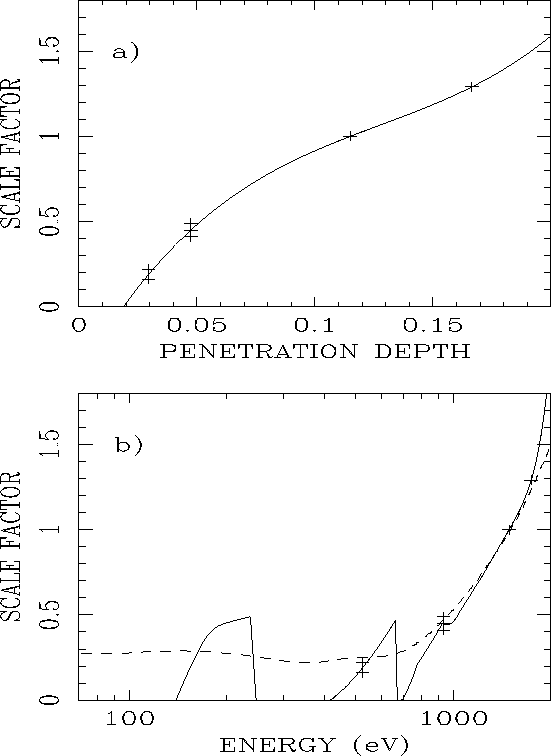Figure 3.10: Amplitude of the high frequency term in the spatial gain correction as a function of penetration depth (a) and energy (b). The plus signs show the data of PSPC-A through D, the solid curve is a third-order polynomial fit to the data. The dashed line in b) shows the curve weighted by effective area, which is used by SASS. The curve is truncated at 1.8. [Hasinger and Snowden1990].

During ground calibrations, the spatial gain variations were measured by illuminating a slit mask which replaced the PSPC entrance window.   Two slit-mask geometries were used: perpendicular and parallel to the anode wires. It was found that perpendicular to the direction of anode A1, the variation is about%, whereas parallel to A1, there is virtually no spatial gain variation (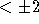%). The magnitude of this effect is a function of the depth within the counter where the photon is absorbed, and therefore of energy (Fig. 3.10).   These measurements were used to construct correction tables to be used for each individual event. (A description of these measurements can be found in [Briel et al.1988]). After correction, tests with an illuminated pinhole mask across the surface of the PSPC revealed a residual gas gain variation of less than 1%, which was consistent with the statistical uncertainty in the mean of the pinhole pulse height spectra.  After correction, the pulse height distribution of a cosmic X-ray source is therefore essentially independent of where within the detector the X-rays were absorbed.

In SASS, this correction is performed by the subroutine DCORG. This correction removes the above described gain variations.  Since the nonlinearities are perpendicular to the anode wire direction, this correction depends on the X coordinate. The correction is parameterized in terms of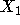, the electronically corrected coordinate system.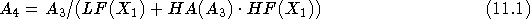where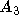are intermediate-processing channels  where PHA channels  have been corrected for ADC nonlinearities, gain-saturation, and temporal variations andare the PI channels   which appear in the events file, and the spatial corrections LF and HF are read from an 800 element vector under (SASS)
` P\$MANPAR:gain_kor3_c.dat` and `gain_kor3_b.dat`
while HA is a 256 vector read from
` P\$MANPAR:gnampl_new.dat`.
The 800 element spatial correction is averaged every 10 detector bins (rounding down) and the spectral vector correction for channels >256, is set to that for channel 256.Next: ADC Non-linearities Up: PSPC Gain Previous: Method of Determination

If you have problems/suggestions please send mail to rosat_svc@mpe-garching.mpg.de